# Array Formulas in Microsoft Excel

An array can be defined as any grouping of two or more adjacent cells. These cells form a square or rectangle. When a selection is defined as an array, operations called array formulas can be performed on every cell in the selection rather than on just a single cell. This is a powerful technique that can be used to enhance Excel formulas and functions.

#### Using Array Formulas

###### Consider the sample worksheet below: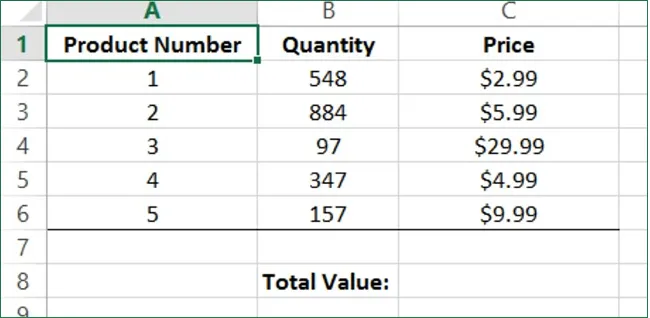If you wanted to calculate the total value of everything in the inventory, you could calculate the value of each quantity and then add all the values together; however, a simpler solution to this problem is to use an array formula.

Click to select cell C8 as this is where the formula will be placed: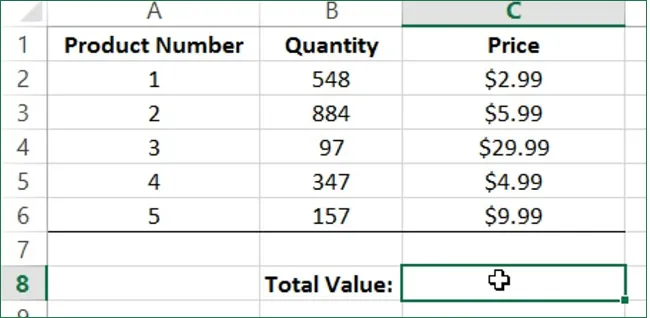This example requires that you multiply one column of values by the other and add all of these results together. With this in mind, type “=SUM(B2:B6*C2:C6)” into the formula bar:While each array is treated as a single argument within the formula, the formula in its current form will result in a #VALUE error. This is because Excel does not recognize the arrays as arguments of the SUM formula.

Correct this by clicking inside the formula bar and pressing Ctrl + Shift + Enter. This action will add curly braces ({}) to both ends of the formula: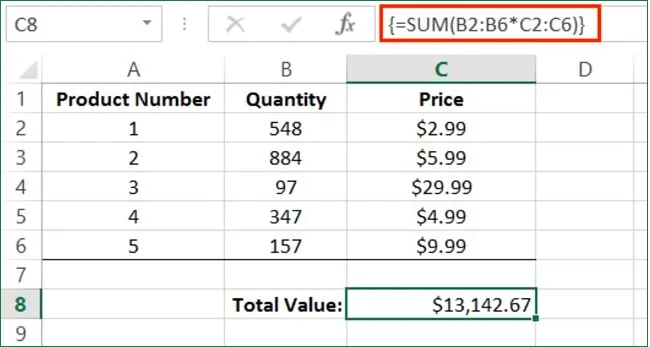These curly braces denote that this formula is an array formula and Excel will treat it as such. You can now see the value of all the stock as determined by the array formula that you just configured: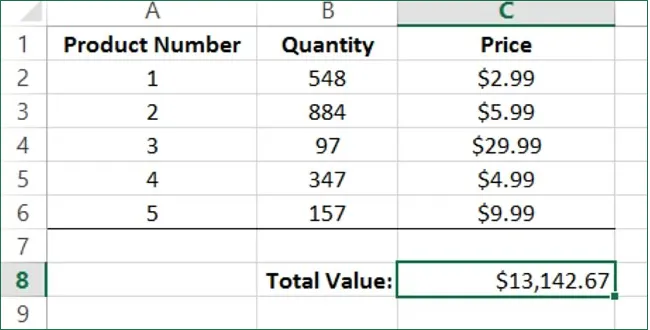Now, suppose that you need to calculate the individual final unit price for each product so that it includes a 5% sales tax. Select cells D2 - D6: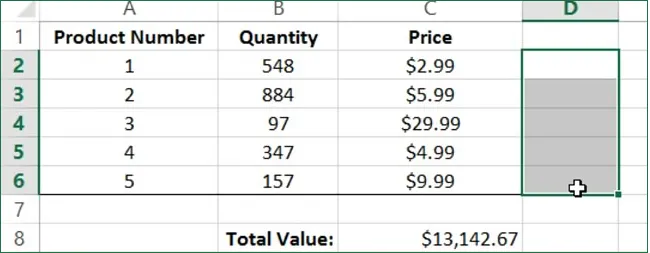In the formula bar, type “=C2:C6*1.05” and then press Ctrl + Shift + Enter: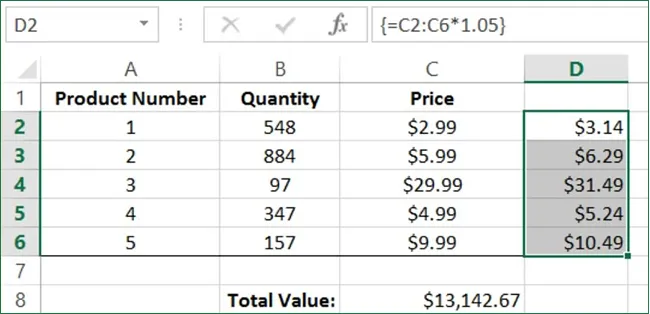As you can see, each value in the first array (C2:C6) has been calculated according to the formula and the result is entered into the corresponding matching place in the second array (D2:D6).

Keep in mind that if you want to edit an array formula, you can change the operators or values in the same manner as any other formula, but you still must press Ctrl + Shift + Enter when you are done to reapply the array formula status.

Save the changes that you have made to the current workbook and then close Microsoft Excel 2013.

#### Onsite Excel training

Through our network of local trainers we deliver onsite Microsoft Excel throughout the country. View our Excel class testimonials or obtain a quote for onsite Excel training.